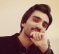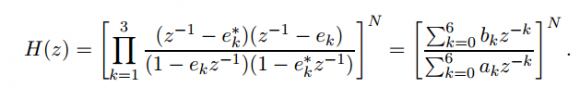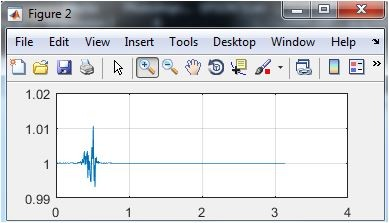## Simulating higher order all-pass filters in matlab?Started by 4 years ago24 replieslatest reply 4 years ago581 views

Hi everyone. I am attempting to implement a higher order all pass filter of the formin MATLAB. The filter coefficients b and a are given by

b = [0.5314, -3.1345, 8.1302, -11.7770, 10.0373, -4.7774, 1.0000]

a = [1.0000, -4.7774, 10.0373, -11.7770, 8.1302, -3.1345, 0.5314]

Using freqz we get a plot of the magnitude response as shown below (for N = 1)Now I have to use N = 50. I tried to convolve b and a vectors with themselves and it gave me a plot like this, which is obviously not all pass.I am making a blunder, some idiotic mistake but I don't know what it is. Any help pointing me in the right direction would be much appreciated. Thanks.

Best regards

Shahbaz A. Khan

BTW I am using the following function to raise b and a to the Nth power.

function y = poly_power(x,N)
% y = poly_power(x,N)
% x is a row vector containing the coefficients of input polynomial
% N is a scalar representing the power to which x is to be raised
% y contains the coefficients of the polynomial y representing x raised...
% ...to the power N

i = 1;
y = x;
while(i<N)
y = conv(y,x);
i = i+1;
end

[ - ]One of the best ways to find a dumb mistake is to answer some dumb questions (I haven't got a clue what you want to do, so I'm the one asking the dumb questions.)  Why are you using convolution?  If you take N=2, don't you get taps out to 12?  How does the conv() operator get that?

Mike

[ - ]The reason I used convolve is that say I have a polynomial (x+1) and I wanna raise it to the second power, then the way to do that is to convolve x with itself. Since in the problem, b and a are raised to the Nth powers so I'm doing convolution N times.

[ - ]First, I'm almost certain that convolving a with b is not the way to go.

Second, Matlab should have a set of functions that will take a ratio of polynomials and build a filter out of it which you can then use to make Bode plots or get time responses.

Third, never, ever make an IIR filter with more than second-order sections.  Separate your 50th-order filter into 25 2nd-order filters and cascade them.  The locations of the roots of polynomials gets ever-more sensitive to coefficient variation as their order goes up; this is reflected in the performance of higher-order IIR filters.  Matlab should have a way of expressing cascaded IIR filters in a way that doesn't run afoul of this effect (I know that Scilab does; I don't know if Matlab does outside of one of their toolboxes).

[ - ]Hi szak1592. Convolving your 'b' coefficients (raising the 'b' coeffs to the Nth power) and convolving your 'a' coefficients (raising the 'a' coeffs to the Nth power) seems OK to me. I performed various orders of convolution and plotted the results' freq magnitude responses. Below is for N = 1 (no convolution of 'b' or 'a'):The freq axis is zero -to- pi radians.

Below is for N = 2 (one convolution of 'b' and 'a'):Below is for N = 3 (two convolutions of 'b' and 'a'):Below is for N = 4 (three convolutions of 'b' and 'a'):Below is for N = 5 (four convolutions of 'b' and 'a'):So after just a few convolutions of the 'b' and 'a' coefficients the resulting freq magnitude response varies noticeably from an ideal allpass filter.

szak1592, I don't know what is the problem here but I am suspicious of the validity (the correctness) of your original 'b' and 'a' coefficients.

Are the magnitudes of your poles and zeros (on the z-plane) EXACT reciprocals of each other? They should be.

[ - ]Hi Rick,

you can plot poles/zeros:

zplane(b,a);

[z,p,k]=tf2zp(b,a)

z =

0.9946 + 0.5888i
0.9946 - 0.5888i
1.0348 + 0.4498i
1.0348 - 0.4498i
0.9199 + 0.5102i
0.9199 - 0.5102i

p =

0.8314 + 0.4611i
0.8314 - 0.4611i
0.7445 + 0.4407i
0.7445 - 0.4407i
0.8128 + 0.3533i
0.8128 - 0.3533i

k =

0.5314

[ - ]Hi kaz. Yes, you're right & I'm familiar with that 'tf2zp(b,a)' command. I wanted szak1592 to determine if his pole and zero magnitudes were EXACT reciprocals of each other, which they are not. I used the following commands:

[z,p,k]=tf2zp(b,a);
format long
format short

His pole and zero magnitudes are very close to being reciprocals of each other, but they are NOT exact reciprocals of each other. So this means szak1592's original filter is very close to being an allpass filter but it is NOT a perfect, ideal, allpass filter.

So, ...cascading his original imperfect filter reveals his original filter's very small imperfection. (Hannibal Lector, Sherlock Holmes, and Lt. Columbo would have figured this out faster than I did.)

[ - ]So the small imperfections add up when cascading and that's what causing the problems. But I'll see if dividing the filter into second order sections still works.

[ - ]Hi Rick,

I did the same before posting the problem here. I tried N = 2 and N = 3 and things were okay but when I tried N = 10, I got crazy results.

The coefficients b and a are from MIT's discrete time signal processing's course project (link below) so I'm guessing the b's and a's are fine.

Something else seems to be the problem.

[ - ]Hi szak1592 (Shahbaz). I'm replying to your "I did the same before ..." post.

If you run the code I listed in my above reply to kaz, and carefully compare the least-significant digits of the 'Zero_Radii' and 'Pole_Radii_Recip' vectors, you will see that the numerical values in those vectors are NOT exactly equal. This means your original filter's poles and zeros are NOT exact reciprocals of each other. Because ideal allpass filter pole and zero magnitudes are EXACT reciprocals of each other, your MIT-generated coefficients NOT perfect! Those MIT-generated coefficients are very very close to being allpass filter coefficients but they are NOT exactly, perfectly, flawlessly, infinitely-precise allpass filter coefficients.

And those coefficents' imperfection IS the main problem here. I think another problem is attempting to model fifty 6th-order IIR filters cascaded with each other. I don't think MATLAB has the numerical precision to accurately perform such modeling.

[ - ]Hi Rick,

But this imperfection would mean that even designing second order sections and cascading them won't work because the sections would have to be convolved ultimately. May be I'll get it to work (fingers crossed).

[ - ]So combining the other answers so far, the first step would be to break up your original filter into 3 cascaded second order filters.  Then cascade 50 of those.  That gives you 150 second order filters.  This should be correct as your original form has a 6th order to the 50th power = 300 taps.  Convolution does not do that.

Your original form already does this actually.  It is a product of 3 second order forms.  What are e_k and e_k^*?  Matlab should be able to derive the complete set of coefficients directly.  And as shown, you should check for N=2, N=3, ... and make sure you're feeding Matlab the right numbers.

[ - ]Hi Mike,

So it seems that is the only thing left to try. I'll try to do that and see if it works.

[ - ]Hi szak1592. Just out of curiosity, can you tell me what is the purpose of cascading 50 (fifty) 6th-order IIR allpass filters? Thanks. (I'm willing to bet one bottle of Czechoslovakian pilsner beer that MATLAB's numerical precision is not great enough to model such process.)

[ - ]I am just attempting this DSP semester project on MIT's site (I mentioned it in one of my replies above) and one of the tasks is to have such a filter and notice the effects of the group delay on a speech sample.

[ - ]Hi szak1592 (Shahbaz). I'm replying to your "I am just attempting this DSP ..." post.

I looked at page 3 of the MIT PDF file and, sure enough, MIT's Project I Part A tells you to cascade fifty allpass filters. (To quote Lt. Joe Kenda, "Well my my my.")

Instead of working on my bathroom sink drain that has been clogged for the last two days I'm going to see if I'm able to cascade fifty IIR allpass filters using MATLAB. I'll keep you informed of my progress.

[ - ]Hi Rick,

It seems like I have figured it out (OR made a fool of myself, I'll let the grown-ups decide).

As suggested by drmike, I used MATLAB's second order sections function to get 150 sections of 2nd order. And seems like using this method, the magnitude response is all good, just the group delay is increased (as it should).

And when I filter the speech signal provided with the project, there is audible distortion when N = 50 is used.

Here is the group delay and magnitude response with N = 50Assuming this is correct, the problem with this method is that I don't know how MATLAB filtered it using the command "sosfilt" (see code below). Maybe you will do another way (if you decided to delay the fixing of the sink drain).

% build a N = 50 order filter by using second order sections
% first divide original filter into 3 second order sections
[z,p,k] = tf2zp(b,a);
sos_1 = zp2sos(z,p,k);

% now get the 50th order filter
N = 50;
sos_50 = [];
for ind = 1:N
sos_50 = [sos_50;sos_1];
end
% view the magnitude response and group delay
fvtool(sos_50)

% filter using the 50th order filter and listen
yy = sosfilt(sos_50,speech);

[ - ]Hi szak1592 (Shahbaz). I am replying to your "It seems like I have figured..." post.

Using your original 6th-order 'b' and 'a' allpass coefficients, when I tried to implement a cascaded filter with N = 4 the resulting 24th-order allpass filter had many poles lying outside the unit circle. (So using such a 24th-order filter is a "train ride the Error City.")

Thinking your original 6th-order 'b' and 'a' coefficients were "defective" in some way, I designed my own 6th-order allpass filter. I did that by converting a chebyshev type-1 IIR filter to an allpass filter using the "pole interlacing property" described on page 89 of Vaidyanathan's DSP book.

Next, using my 6th-order 'b' and 'a' allpass coefficients, when I tried to implement a cascaded filter with N = 4 the resulting 24th-order allpass filter also had many poles lying outside the unit circle. As with your original coefficients, my 6th-order filter's coefficients have pole and zero magnitudes that are NOT exact reciprocals of each other.

So now I no longer think your original 6th-order 'b' and 'a' coefficients are defective in any way. (So there's no reason for you to call your attorney and file a class action lawsuit against MIT.) What I've demonstrated here is a numerical precision problem and why people do not implement super high-order IIR filters. So my next question is, How do the MIT guys expect you to "Implement the filter for N=50"?

Dr. mike's and your suggestion of breaking your 6th-order filter into 2nd-order sections is certainly a good idea. I will experiment with that idea. (My sink is still clogged.)

[ - ]Hi Rick,

At least there's something new I learned from all this, esp. since you experimented with other b's and a's and still ended up with the same problem when cascading using polynomial convolution. So thank you.

Because I wanna implement this pole interlacing property myself, what DSP book is this? Vaidyanathan's caltech page says he has written 4 books?

And BTW, if you do play around with this idea of breaking into second order sections, please do let me know how you did it and how it compared to the way I did it. (If household chores permit :) ).

[ - ]Hi szak1592 (Shahbaz). I'm replying to your "At least there's something ..." post.

 Here's my frequency response analysis of your original 'b' and 'a' coefficients:

%%%%%%%%%%%%%%%%%%%%%%%%%%%%%%%%%%%%%%%%%%%%%%%%
% Lyons' Frequency Response Analysis
%%%%%%%%%%%%%%%%%%%%%%%%%%%%%%%%%%%%%%%%%%%%%%%%%%
[z,p,k] = tf2zp(b,a);
sos_1 = zp2sos(z,p,k);
b1 = sos_1(1, 1:3); a1 = sos_1(1, 4:6);
b2 = sos_1(2, 1:3); a2 = sos_1(2, 4:6);
b3 = sos_1(3, 1:3); a3 = sos_1(3, 4:6);
N = 50;

[H1, W] = freqz(b1, a1, 256);
[H2, W] = freqz(b2, a2, 256);
[H3, W] = freqz(b3, a3, 256);
H1_Phase = unwrap(angle(H1));
H2_Phase = unwrap(angle(H2));
H3_Phase = unwrap(angle(H3));
H1_conv = H1.^N; H2_conv = H2.^N; H3_conv = H3.^N;
H_total = H1_conv.*H2_conv.*H3_conv;
H_total_mag = abs(H_total);
H_total_dB = 20*log10(H_total_mag);
H_total_Phase_1 = unwrap(angle(H_total));
H_total_Phase_2 = N*H1_Phase + N*H2_Phase + N*H3_Phase;
Freq = W/(2*pi);

figure(4), clf
subplot(2,1,1)
plot(Freq, H_total_mag); ylabel('Lin.'), grid on, zoom on
subplot(2,1,2)
plot(Freq, abs(H_total_dB)); ylabel('dB'), grid on, zoom on

figure(5), clf
subplot(2,1,1)
plot(Freq, H_total_Phase_1); ylabel('Phase-1'), grid on, zoom on
subplot(2,1,2)
plot(Freq, H_total_Phase_2); ylabel('Phase-2'), grid on, zoom on
%%%%%%%%%%%%%%%%%%%%%%%%%%%%%%%%%%%%%%%%%%%%%%%%%%%

What's super-interesting to me is that my 'Phase_1' method for computing the phase of the N=50 filter gives erroneous results! That's why I used my 'Phase_2' method for computing the phase of the N=50 filter which computes a phase response result equal to your fvtool() command's phase response.

Your use of the fvtool() command was a smart thing to do. My playing around here has been both educational and fun for me.

 The Vaidyanathan book I used was his "Multirate Systems and Filter Banks" book. (A book that's difficult to read but it contains a truly astounding amount of DSP theory and information.)

 Shahbaz, months ago I starting working on a blog discussing how make IIR filter implementations more efficient using allpass filters. That blog is a bit complicated so I have not yet finished it. In any case, in that blog I intend to present my MATLAB code for using the "pole interlacing property" to compute allpass filters. (By the way, Sanjit Mitra's terrific "DSP, a Computer-Based Approach" book also discusses the "pole interlacing property".)

 The sink is still clogged.

[ - ]Hi. In an earlier post of mine I wondered, "How do the MIT guys expect you to "Implement the filter for N=50"?" I can think of two ways to do that:

 Compute the impulse response (IR) of the original 6th-order IIR filter. Convolve that IR with itself 49 times to compute an "N=50 IR". Then convolve input signal with the "N=50 IR".

 Pass the input signal through the original 6th-order IIR filter. Then pass the filter's output through the original 6th-order IIR filter. Do that 49 more times.

[ - ]Good luck with that sink!!! :-)  And thanks for the lessons.

[ - ]Hi Dr. Mike. I hope all is well with you!

[ - ]